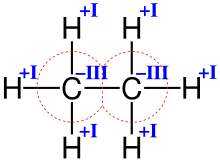91 Pages

## The BasicsEdit

Oxidation Numbers are a little like the charge on an ion of an element.

So the Oxidation Number of Calcium in Ca2+ is +2.In reality, neither the Carbon nor Hydrogen atoms have actually completely gained or lost electrons.

And the Oxidation Number of Fluorine in F- is -1.

But the Oxidation Number of both Calcium metal Fluorine gas is 0 because elements retain control of all of their own electrons.

But the Oxidation Number of Chlorine in ClO3- is +5 - this doesn't really mean that the Chlorine atom has lost 5 electrons.

In reality, the Chlorine atom has donated 5 electrons to the 6 bonds. The Oxygen atoms have donated six electrons, leaving one spare for the 1- charge.

Neither element has actually lost the electrons but Oxygen atoms are more electronegative so they have partially gained one electron each (giving an Oxidation Number of -1) and the Chlorine atom has partially lost 5 electrons (hence +5).

In reality, Oxidation Numbers are just a handy way of seeing which substances have been Oxidised or Reduced.

An increase in Oxidation Number is Oxidation: a decrease in Oxidation Number is Reduction.

## The RulesEdit

All elements have an Oxidation Number of 0.

Group I elements only form compounds where they have Oxidation Number = 1

Group II elements only form compounds where they have Oxidation Number = 2.

Oxygen generally forms compounds where it has Oxidation Number = -2. (In Peroxides it is -1).

Hydrogen generally forms compounds where it has Oxidation Number = +1. (In Metal Hydrides it is -1).

Fluorine always forms compounds where it has Oxidation Number = -1.

## Applying the RulesEdit

What is the Oxidation Number of Sulphur in Sulphur Dioxide?

SO2

2 x Oxygen (-2) = -4

So the sulphur must be +4 to balance the -4.

What is the Oxidation Number of Sulphur in Sulphuric Acid?

H2SO4

2 x Hydrogen (+1) = +2

4 x Oxygen = -8

This makes a total of -6.

Overall, H2SO4 has no charge so the Sulphur must be +6 to balance the -6.

What is the Oxidation Number of Sulphur in SO32-?

SO32-

3 x Oxygen (-2) = -6

This makes a total of -6 but there is a 2- charge left over.

So the Sulphur isn't cancelling all the -6 charge and must only be +4. ( -6 + 4 = -2 )